# Visualizing Evoked data¶

This tutorial shows the different visualization methods for `Evoked` objects.

As usual we’ll start by importing the modules we need:

```import os
import numpy as np
import mne
```

Instead of creating the `Evoked` object from an `Epochs` object, we’ll load an existing `Evoked` object from disk. Remember, the `.fif` format can store multiple `Evoked` objects, so we’ll end up with a `list` of `Evoked` objects after loading. Recall also from the Loading and saving Evoked data section of the introductory Evoked tutorial that the sample `Evoked` objects have not been baseline-corrected and have unapplied projectors, so we’ll take care of that when loading:

```sample_data_folder = mne.datasets.sample.data_path()
sample_data_evk_file = os.path.join(sample_data_folder, 'MEG', 'sample',
'sample_audvis-ave.fif')
proj=True, verbose=False)

# Show the condition names, and reassure ourselves that baseline correction has
# been applied.
for e in evokeds_list:
print(f'Condition: {e.comment}, baseline: {e.baseline}')
```

Out:

```Condition: Left Auditory, baseline: (-0.19979521315838786, 0.0)
Condition: Right Auditory, baseline: (-0.19979521315838786, 0.0)
Condition: Left visual, baseline: (-0.19979521315838786, 0.0)
Condition: Right visual, baseline: (-0.19979521315838786, 0.0)
```

To make our life easier, let’s convert that list of `Evoked` objects into a `dictionary`. We’ll use `/`-separated dictionary keys to encode the conditions (like is often done when epoching) because some of the plotting methods can take advantage of that style of coding.

```conds = ('aud/left', 'aud/right', 'vis/left', 'vis/right')
evks = dict(zip(conds, evokeds_list))
#      ‾‾‾‾‾‾‾‾‾‾‾‾‾‾‾‾‾‾‾‾‾‾‾‾‾‾‾‾‾‾ this is equivalent to:
# {'aud/left': evokeds_list, 'aud/right': evokeds_list,
#  'vis/left': evokeds_list, 'vis/right': evokeds_list}
```

## Plotting signal traces¶

The most basic plot of `Evoked` objects is a butterfly plot of each channel type, generated by the `evoked.plot()` method. By default, channels marked as “bad” are suppressed, but you can control this by passing an empty `list` to the `exclude` parameter (default is `exclude='bads'`):

```evks['aud/left'].plot(exclude=[])
```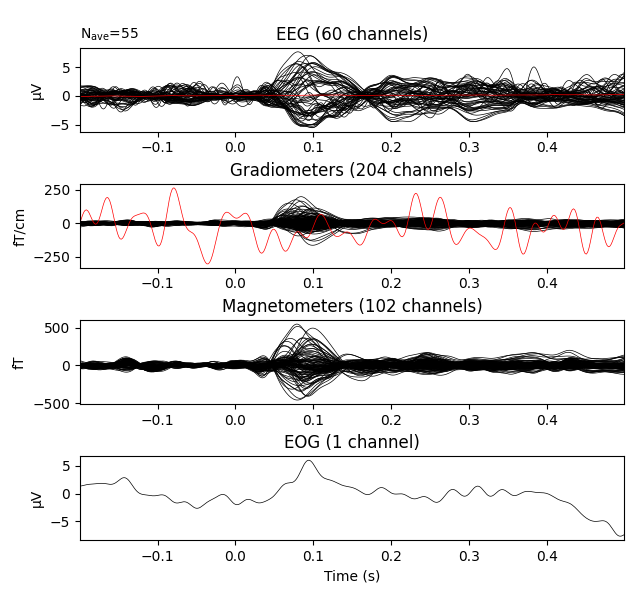Notice the completely flat EEG channel and the noisy gradiometer channel plotted in red color. Like many MNE-Python plotting functions, `evoked.plot()` has a `picks` parameter that can select channels to plot by name, index, or type. In the next plot we’ll show only magnetometer channels, and also color-code the channel traces by their location by passing `spatial_colors=True`. Finally, we’ll superimpose a trace of the root mean square (RMS) of the signal across channels by passing `gfp=True`. This parameter is called `gfp` for historical reasons and behaves correctly for all supported channel types: for MEG data, it will plot the RMS; while for EEG, it would plot the global field power (an average-referenced RMS), hence its name:

```evks['aud/left'].plot(picks='mag', spatial_colors=True, gfp=True)
```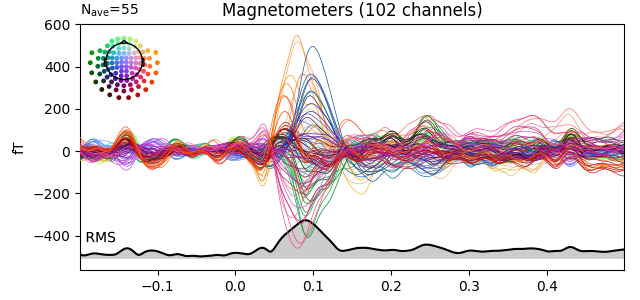## Plotting scalp topographies¶

In an interactive session, the butterfly plots seen above can be click-dragged to select a time region, which will pop up a map of the average field distribution over the scalp for the selected time span. You can also generate scalp topographies at specific times or time spans using the `plot_topomap` method:

```times = np.linspace(0.05, 0.13, 5)
evks['aud/left'].plot_topomap(ch_type='mag', times=times, colorbar=True)
```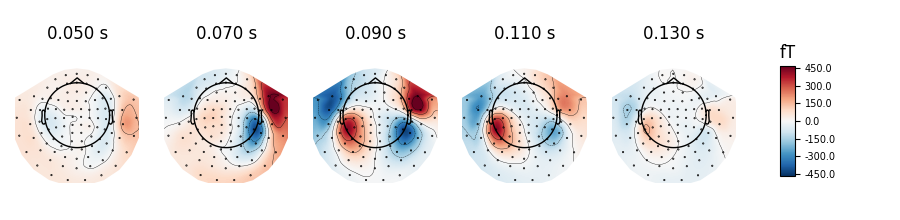Out:

```Removing projector <Projection | Average EEG reference, active : True, n_channels : 60>
```
```fig = evks['aud/left'].plot_topomap(ch_type='mag', times=0.09, average=0.1)
fig.text(0.5, 0.05, 'average from 40-140 ms', ha='center')
```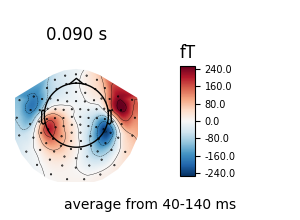Out:

```Removing projector <Projection | Average EEG reference, active : True, n_channels : 60>
```

Additional examples of plotting scalp topographies can be found in Plotting topographic maps of evoked data.

## Arrow maps¶

Scalp topographies at a given time point can be augmented with arrows to show the estimated magnitude and direction of the magnetic field, using the function `mne.viz.plot_arrowmap`:

```mags = evks['aud/left'].copy().pick_types(meg='mag')
mne.viz.plot_arrowmap(mags.data[:, 175], mags.info, extrapolate='local')
```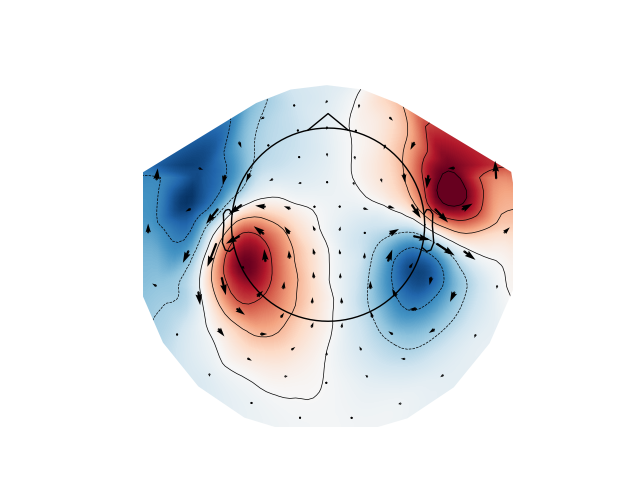## Joint plots¶

Joint plots combine butterfly plots with scalp topographies, and provide an excellent first-look at evoked data; by default, topographies will be automatically placed based on peak finding. Here we plot the right-visual-field condition; if no `picks` are specified we get a separate figure for each channel type:

```evks['vis/right'].plot_joint()
```
•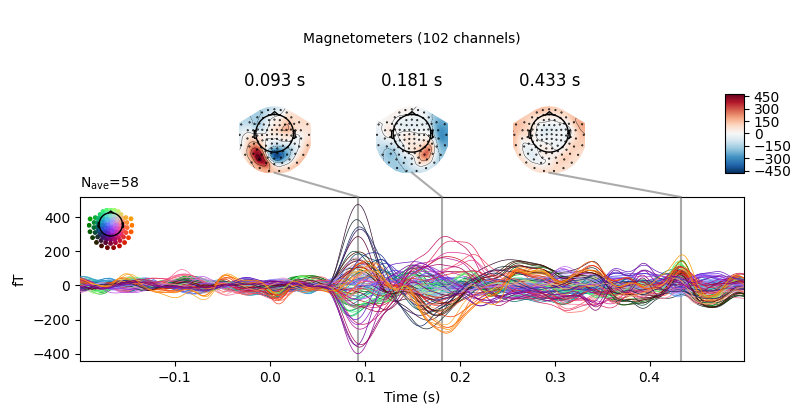•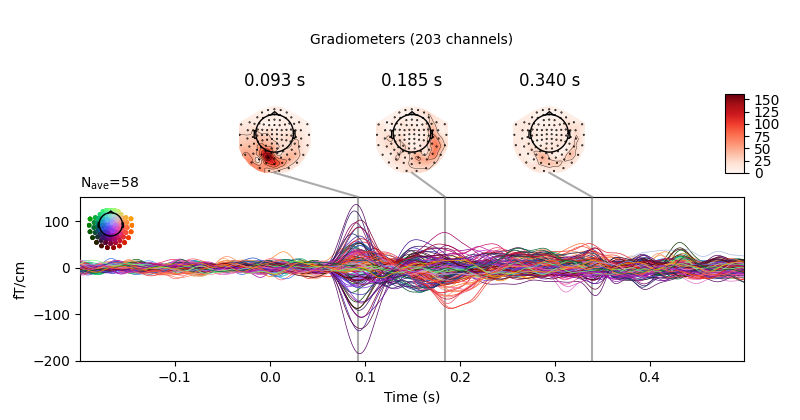•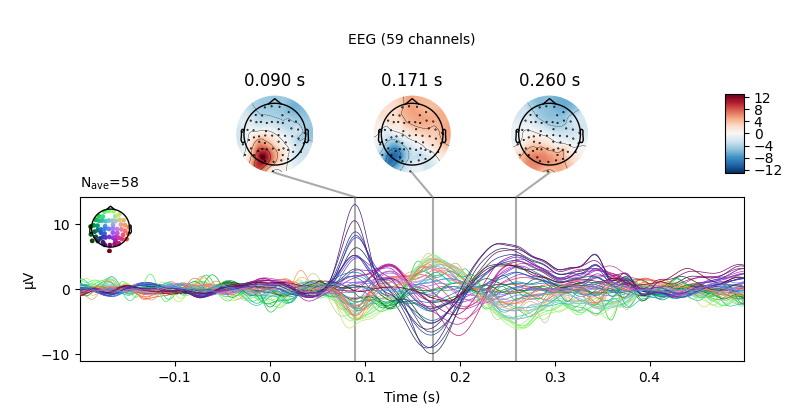Out:

```Removing projector <Projection | Average EEG reference, active : True, n_channels : 60>
Removing projector <Projection | PCA-v1, active : True, n_channels : 102>
Removing projector <Projection | PCA-v2, active : True, n_channels : 102>
Removing projector <Projection | PCA-v3, active : True, n_channels : 102>
Removing projector <Projection | Average EEG reference, active : True, n_channels : 60>
Removing projector <Projection | PCA-v1, active : True, n_channels : 102>
Removing projector <Projection | PCA-v2, active : True, n_channels : 102>
Removing projector <Projection | PCA-v3, active : True, n_channels : 102>
```

Like `plot_topomap` you can specify the `times` at which you want the scalp topographies calculated, and you can customize the plot in various other ways as well. See `mne.Evoked.plot_joint` for details.

## Comparing `Evoked` objects¶

To compare `Evoked` objects from different experimental conditions, the function `mne.viz.plot_compare_evokeds` can take a `list` or `dict` of `Evoked` objects and plot them all on the same axes. Like most MNE-Python visualization functions, it has a `picks` parameter for selecting channels, but by default will generate one figure for each channel type, and combine information across channels of the same type by calculating the global field power. Information may be combined across channels in other ways too; support for combining via mean, median, or standard deviation are built-in, and custom callable functions may also be used, as shown here:

```def custom_func(x):
return x.max(axis=1)

for combine in ('mean', 'median', 'gfp', custom_func):
mne.viz.plot_compare_evokeds(evks, picks='eeg', combine=combine)
```
•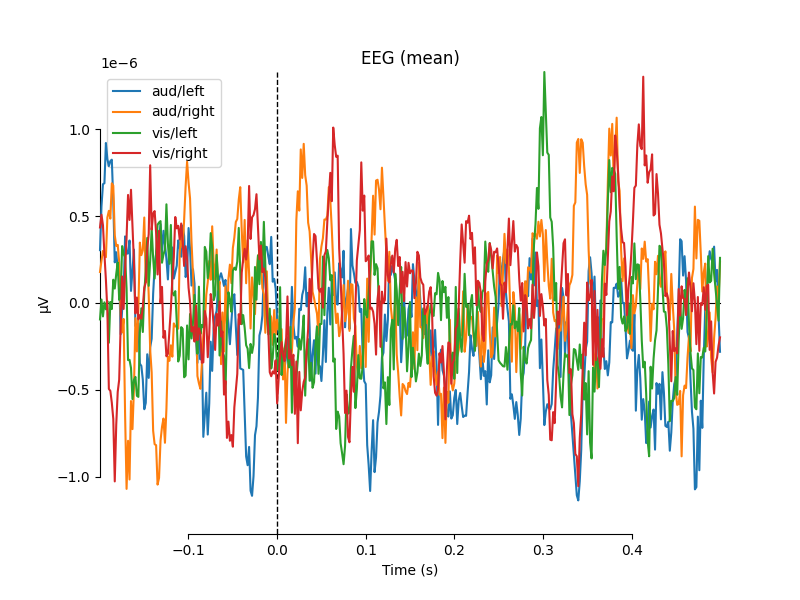•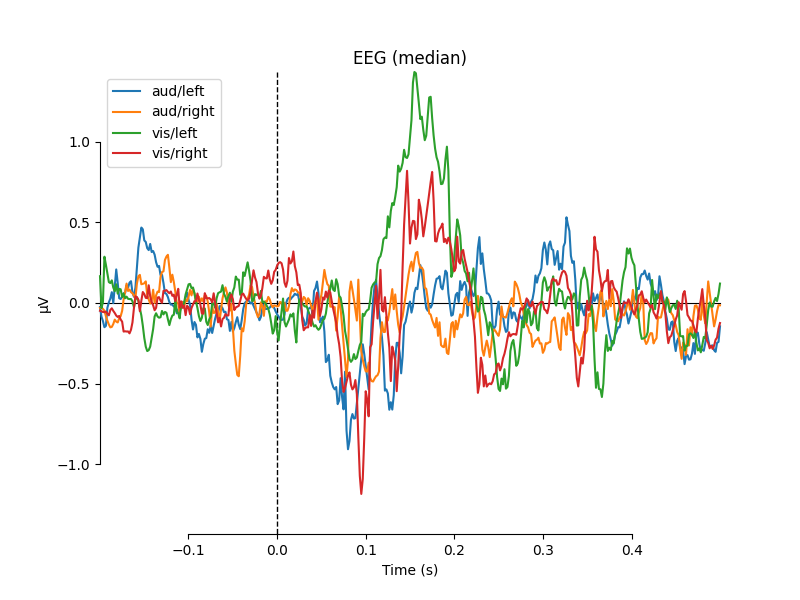•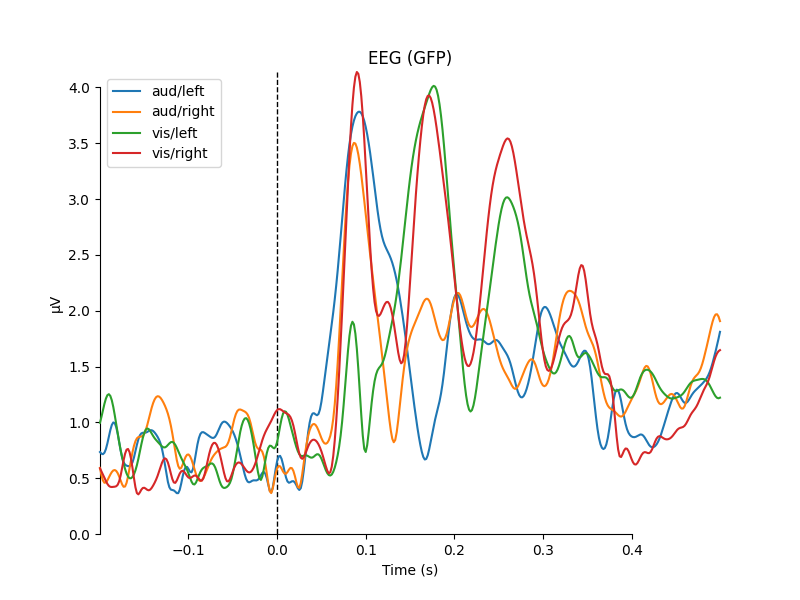•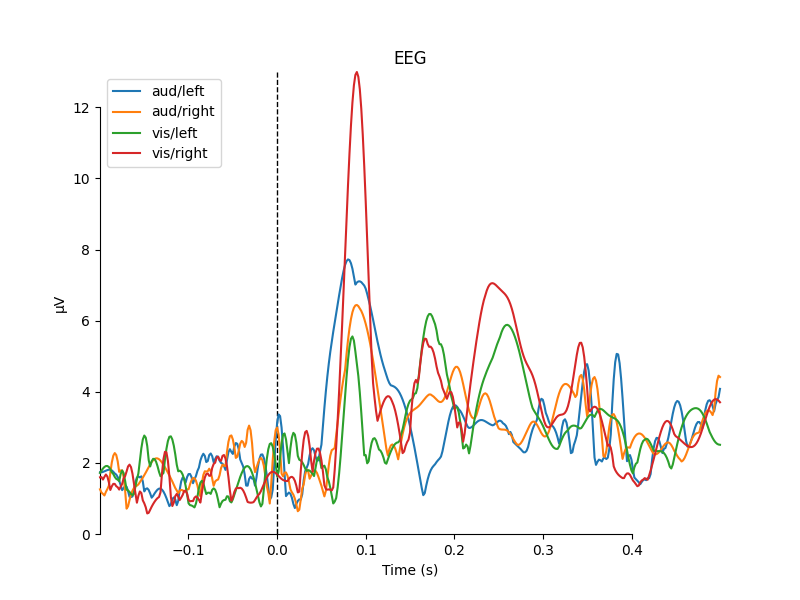Out:

```combining channels using "mean"
combining channels using "mean"
combining channels using "mean"
combining channels using "mean"
combining channels using "median"
combining channels using "median"
combining channels using "median"
combining channels using "median"
combining channels using "gfp"
combining channels using "gfp"
combining channels using "gfp"
combining channels using "gfp"
combining channels using "<function custom_func at 0x7fe8f7935160>"
combining channels using "<function custom_func at 0x7fe8f7935160>"
combining channels using "<function custom_func at 0x7fe8f7935160>"
combining channels using "<function custom_func at 0x7fe8f7935160>"
```

One nice feature of `plot_compare_evokeds` is that when passing evokeds in a dictionary, it allows specifying plot styles based on `/`-separated substrings of the dictionary keys (similar to epoch selection; see Subselecting epochs). Here, we specify colors for “aud” and “vis” conditions, and linestyles for “left” and “right” conditions, and the traces and legend are styled accordingly.

```mne.viz.plot_compare_evokeds(evks, picks='MEG 1811', colors=dict(aud=0, vis=1),
linestyles=dict(left='solid', right='dashed'))
```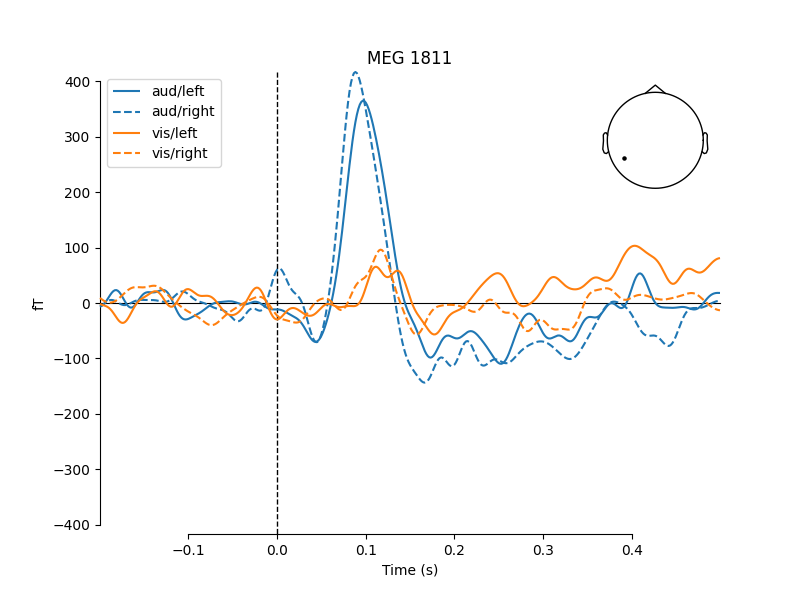The legends generated by `plot_compare_evokeds` above used the dictionary keys provided by the `evks` variable. If instead you pass a `list` or `tuple` of `Evoked` objects, the legend keys will be generated automatically from the `comment` attribute of the `Evoked` objects (or, as sequential integers if the comment attribute is empty or ambiguous). To illustrate this, we’ll make a list of 5 `Evoked` objects: 2 with identical comments, 2 with empty comments (either an empty string or `None`), and 1 with a unique non-empty comment:

```temp_list = list()
for idx, _comment in enumerate(('foo', 'foo', '', None, 'bar'), start=1):
_evk = evokeds_list.copy()
_evk.comment = _comment
_evk.data *= idx  # so we can tell the traces apart
temp_list.append(_evk)

mne.viz.plot_compare_evokeds(temp_list, picks='mag')
```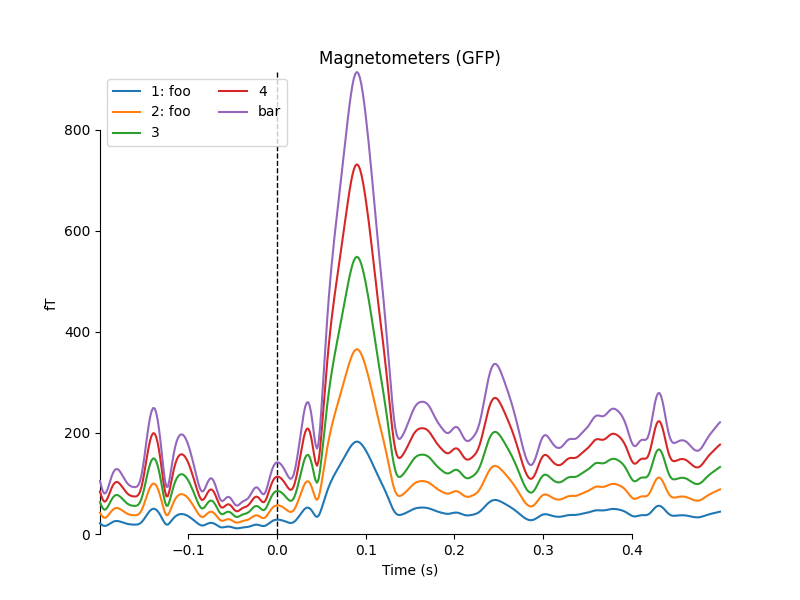Out:

```combining channels using "gfp"
combining channels using "gfp"
combining channels using "gfp"
combining channels using "gfp"
combining channels using "gfp"
```

## Image plots¶

Like `Epochs`, `Evoked` objects also have a `plot_image` method, but unlike `epochs.plot_image()`, `evoked.plot_image()` shows one channel per row instead of one epoch per row. Again, a `picks` parameter is available, as well as several other customization options; see `plot_image` for details.

```evks['vis/right'].plot_image(picks='meg')
```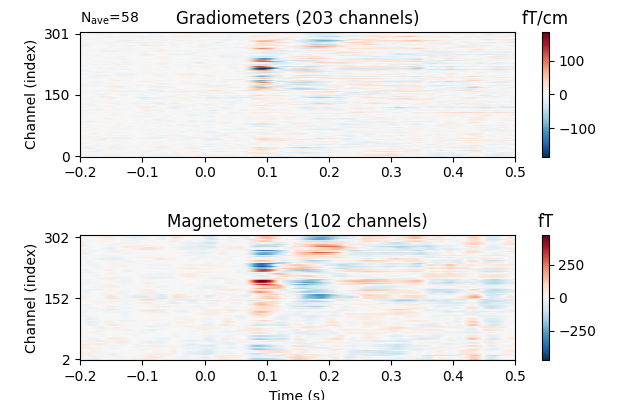## Topographical subplots¶

For sensor-level analyses it can be useful to plot the response at each sensor in a topographical layout. The `plot_compare_evokeds` function can do this if you pass `axes='topo'`, but it can be quite slow if the number of sensors is too large, so here we’ll plot only the EEG channels:

```mne.viz.plot_compare_evokeds(evks, picks='eeg', colors=dict(aud=0, vis=1),
linestyles=dict(left='solid', right='dashed'),
axes='topo', styles=dict(aud=dict(linewidth=1),
vis=dict(linewidth=1)))
```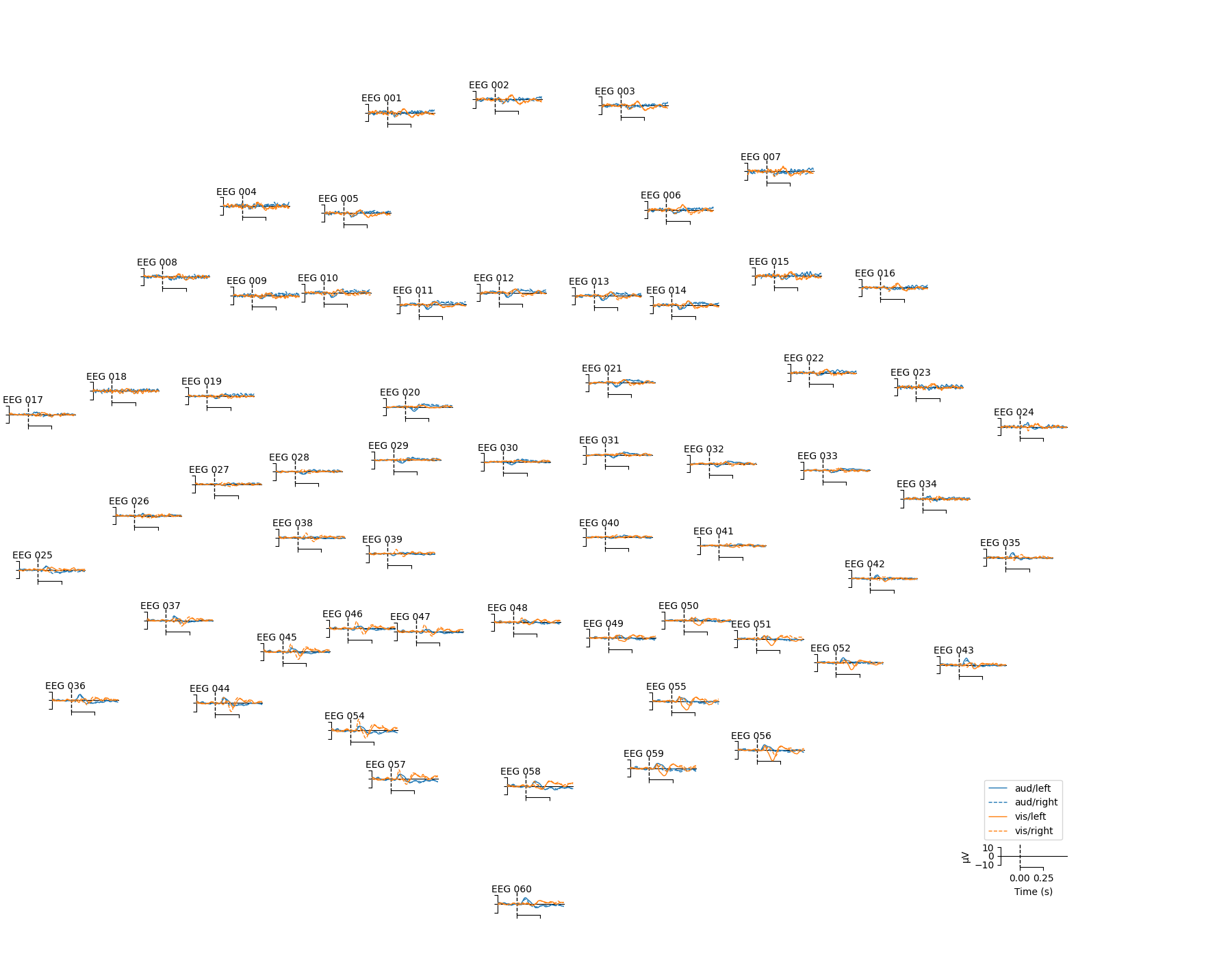For larger numbers of sensors, the method `evoked.plot_topo()` and the function `mne.viz.plot_evoked_topo` can both be used. The `plot_topo` method will plot only a single condition, while the `plot_evoked_topo` function can plot one or more conditions on the same axes, if passed a list of `Evoked` objects. The legend entries will be automatically drawn from the `Evoked` objects’ `comment` attribute:

```mne.viz.plot_evoked_topo(evokeds_list)
```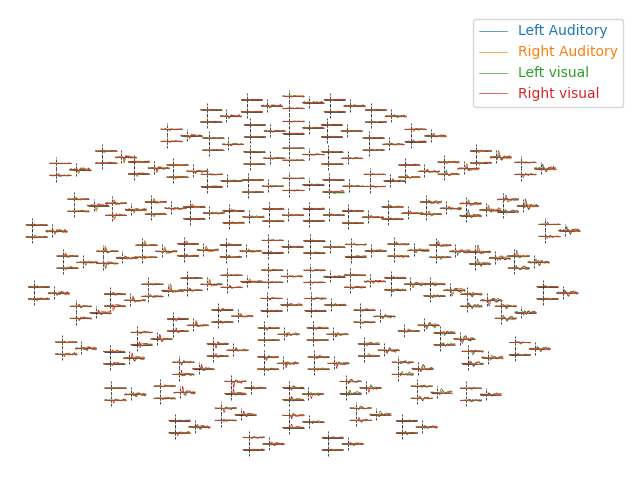By default, `plot_evoked_topo` will plot all MEG sensors (if present), so to get EEG sensors you would need to modify the evoked objects first (e.g., using `mne.pick_types`).

Note

In interactive sessions, both approaches to topographical plotting allow you to click one of the sensor subplots to pop open a larger version of the evoked plot at that sensor.

## 3D Field Maps¶

The scalp topographies above were all projected into 2-dimensional overhead views of the field, but it is also possible to plot field maps in 3D. To do this requires a trans file to transform locations between the coordinate systems of the MEG device and the head surface (based on the MRI). You can compute 3D field maps without a `trans` file, but it will only work for calculating the field on the MEG helmet from the MEG sensors.

```subjects_dir = os.path.join(sample_data_folder, 'subjects')
sample_data_trans_file = os.path.join(sample_data_folder, 'MEG', 'sample',
'sample_audvis_raw-trans.fif')
```

By default, MEG sensors will be used to estimate the field on the helmet surface, while EEG sensors will be used to estimate the field on the scalp. Once the maps are computed, you can plot them with `evoked.plot_field()`:

```maps = mne.make_field_map(evks['aud/left'], trans=sample_data_trans_file,
subject='sample', subjects_dir=subjects_dir)
evks['aud/left'].plot_field(maps, time=0.1)
```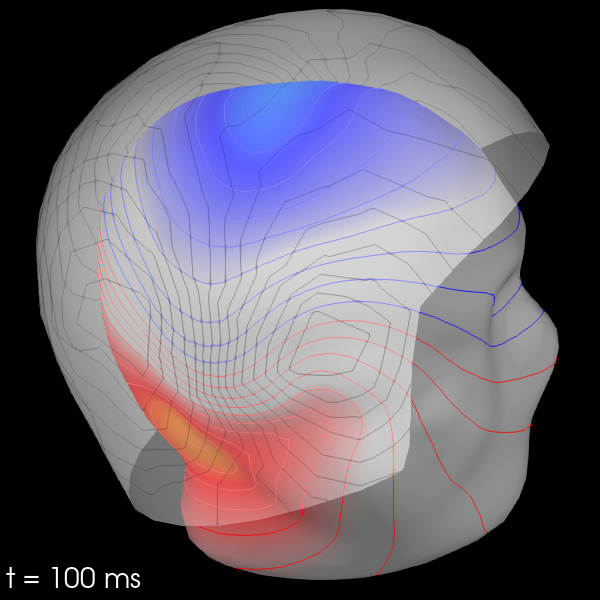Out:

```Using surface from /home/circleci/mne_data/MNE-sample-data/subjects/sample/bem/sample-5120-5120-5120-bem.fif.
Getting helmet for system 306m
Prepare EEG mapping...
Computing dot products for 59 electrodes...
Computing dot products for 2562 surface locations...
Preparing the mapping matrix...
Truncating at 21/59 components to omit less than 0.001 (0.00097)
The map has an average electrode reference (2562 channels)
Prepare MEG mapping...
Computing dot products for 305 coils...
Computing dot products for 304 surface locations...
Preparing the mapping matrix...
Truncating at 210/305 components to omit less than 0.0001 (9.9e-05)
```

You can also use MEG sensors to estimate the scalp field by passing `meg_surf='head'`. By selecting each sensor type in turn, you can compare the scalp field estimates from each.

```for ch_type in ('mag', 'grad', 'eeg'):
evk = evks['aud/right'].copy().pick(ch_type)
_map = mne.make_field_map(evk, trans=sample_data_trans_file,
subject='sample', subjects_dir=subjects_dir,
fig = evk.plot_field(_map, time=0.1)
mne.viz.set_3d_title(fig, ch_type, size=20)
```
•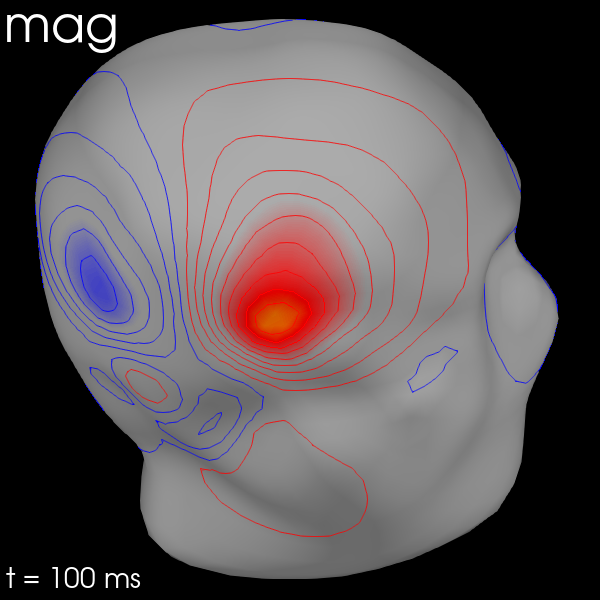•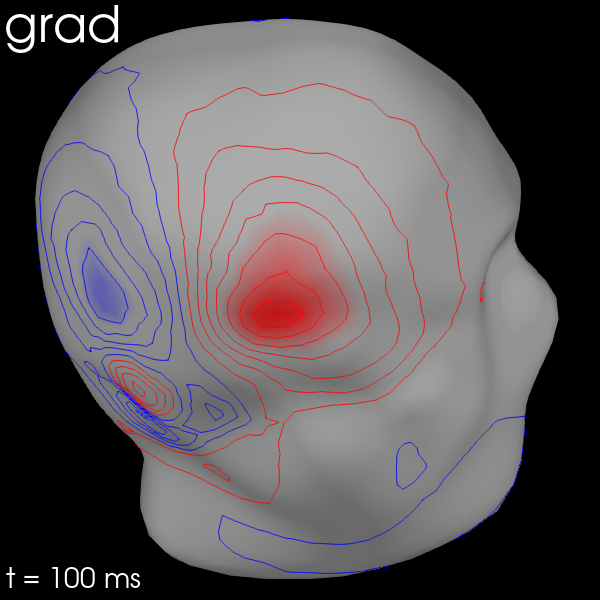•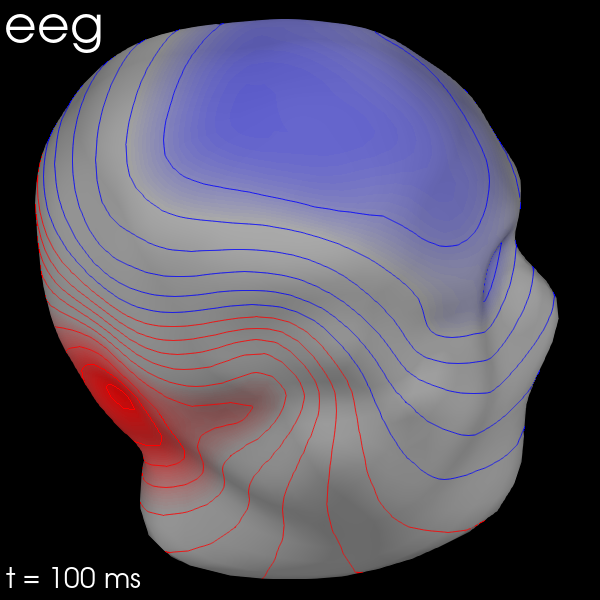Out:

```Removing projector <Projection | Average EEG reference, active : True, n_channels : 60>
Using surface from /home/circleci/mne_data/MNE-sample-data/subjects/sample/bem/sample-5120-5120-5120-bem.fif.
Prepare MEG mapping...
Computing dot products for 102 coils...
Computing dot products for 2562 surface locations...
Preparing the mapping matrix...
Truncating at 57/102 components to omit less than 0.0001 (9.9e-05)
Removing projector <Projection | PCA-v1, active : True, n_channels : 102>
Removing projector <Projection | PCA-v2, active : True, n_channels : 102>
Removing projector <Projection | PCA-v3, active : True, n_channels : 102>
Removing projector <Projection | Average EEG reference, active : True, n_channels : 60>
Using surface from /home/circleci/mne_data/MNE-sample-data/subjects/sample/bem/sample-5120-5120-5120-bem.fif.
Prepare MEG mapping...
Computing dot products for 203 coils...
Computing dot products for 2562 surface locations...
Preparing the mapping matrix...
Truncating at 166/203 components to omit less than 0.0001 (9.7e-05)
Removing projector <Projection | PCA-v1, active : True, n_channels : 102>
Removing projector <Projection | PCA-v2, active : True, n_channels : 102>
Removing projector <Projection | PCA-v3, active : True, n_channels : 102>
Using surface from /home/circleci/mne_data/MNE-sample-data/subjects/sample/bem/sample-5120-5120-5120-bem.fif.
Prepare EEG mapping...
Computing dot products for 59 electrodes...
Computing dot products for 2562 surface locations...